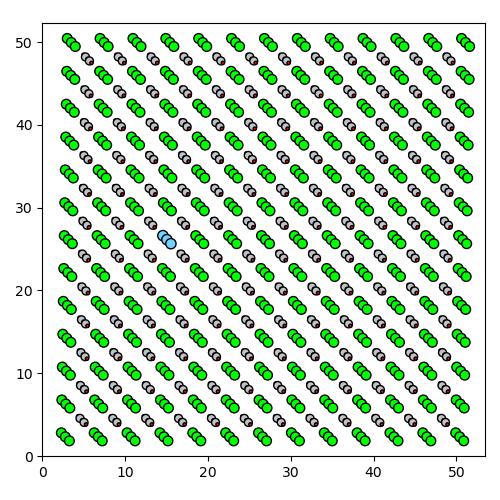# Working with atomic models¶

Atomap provides functions to both export data to atomic models and to import data from atomic models. This functionality is realised through interaction with the Atoms object of the Atomic Simulation Environment (ASE) package.

## Exporting to atomic models¶

`atomap.atom_lattice.Atom_Lattice` objects can be converted to an ASE Atoms object using the `convert_to_ase()` function. Before conversion, all `atomap.atom_position.Atom_Position` objects must have their `element_info` set. The easiest way to set `element_info` for each atom position is to set the same values for all atoms in a `Sublattice`. Both the calibration in the Atom_Lattice and the Z-positions specified with set_element_info needs to be in Ångstrøm. For example:

```>>> import atomap.api as am
>>> atom_lattice = am.dummy_data.get_perovskite_001_atom_lattice(set_element_info=False)
>>> atom_lattice.set_scale(scale=0.2, units="Å")
>>> atom_lattice.sublattice_list.set_element_info('Sr', [0., 4., 8., 12., 16.])
>>> atom_lattice.sublattice_list.set_element_info('Ti', [2., 6., 10., 14.])
>>> atoms = atom_lattice.convert_to_ase()
```

The converted Atoms object can now be saved as an atomic structure file (xyz, cif, etc…), can be visualized in 3D and can be input to atomistic simulations.

Visualization of atomic structure in 3D:

```>>> from ase.visualize import view
>>> view(atoms)
```

If there are different elements in an atomic column, each individual Z-position in an atomic column can be set. For the second sublattice:

```>>> atom_lattice = am.dummy_data.get_perovskite_001_atom_lattice(set_element_info=False)
>>> atom_lattice.set_scale(scale=0.2, units="Å")
>>> atom_lattice.sublattice_list.set_element_info('Sr', [0., 4., 8.])
>>> atom_lattice.sublattice_list.set_element_info(
...        ['O', 'Ti', 'O', 'Ti', 'O'], [0., 2., 4., 6., 8.])
>>> atoms = atom_lattice.convert_to_ase()
>>> view(atoms)
```

This can also be set for individual atoms. For example, lets replace the Strontium column with Lanthanum.

```>>> atom = atom_lattice.sublattice_list.atom_list
>>> atom.set_element_info("La", [0, 4, 8])
>>> view(atoms)
```## Importing atomic models¶

It is also possible to import an atomic model from ASE, to create an `Atom_Lattice` object. To do this, use the `ase_to_atom_lattice()` function. For example, to import a nanoparticle example dataset from ASE:

```>>> import atomap.api as am
>>> from ase.cluster import Octahedron
>>> atoms = Octahedron('Ag', 10, cutoff=2)
>>> atomlattice = am.ase_to_atom_lattice(atoms, (128, 128), gaussian_blur=1)
```